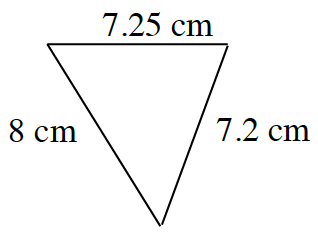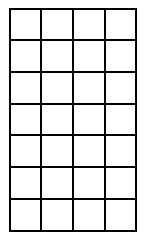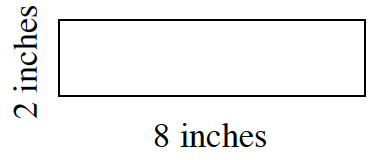### Home > MC2 > Chapter 2 > Lesson 2.1.2 > Problem2-27

2-27.

Find the measure indicated in each question below.

1. Find the perimeter of the triangle.Remember that perimeter is the sum of the border lengths of the shape.

1. Find the area of the large rectangle.Remember that area is the number of square units in a shape.

1. Find the area and perimeter of the rectangle below.See parts (a) and (b).

$\text{Area} = 16 \text{ sq. inches}$
$\text{Perimeter} = 20 \text{ inches}$
Be sure to know how to find these answers using the given measurements.